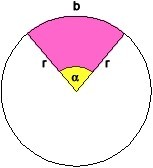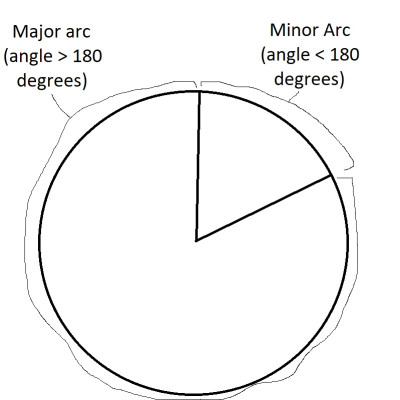# Area Of A Sector Of A Circle (3 Key Concepts To Grasp)

You might already be familiar with finding the area of a circle.  However, finding the area of a sector takes an extra step, since we are finding just a part of the area.

So, what do you need to know about the area of a sector of a circle?  The area A of a sector of a circle is A = R2θ/2, where R is the radius and θ is the angle measure of the arc (in radians). The equivalent formula is A = πR2θ/360 if θ is in degrees. Area of a sector of a circle is a percentage of the entire area, based on the ratio of the angle θ over 2π radians (360 degrees).

Of course, there are various situations when we might need to calculate area of a sector of a circle, including for sectors formed from minor, semicircular, and major arcs.

In this article, we’ll take a closer look at area of a sector of a circle and what it means.  We’ll also look at some examples of how to calculate the area of a sector of a circle in various cases.

Let’s get started.

## Area Of A Sector Of A Circle

The area of a sector of a circle (or circular sector) is a part of the area.  It corresponds to the angle measure of the circular sector.The area of a sector of a circle is a part of the area. It depends on the radius of the circle and the angle measure of the sector.

One way to think of area of a circular sector is how much space there is in that specific part of the circle.  The area of a sector for an entire circle would be the whole area, while the area of a sector for a semicircle would be half of the area.

### Area Formula Of A Sector Of A Circle

The area formula of a sector of a circle depends on whether the angle measure of the arc is given in radians or degrees.The area of a sector of a circle is a part of the area. The part is a fraction that depends on the angle measure.

Area Of A Sector Formula Radians:

• A = R2θ/2, where A is area of the sector, R is radius of the circle, and θ is the angle measure of the arc in radians.

Arc Length Formula Degrees:

• A = πR2θ/360, where A is area of the sector, R is radius of the circle, and θ is the angle measure of the arc in degrees.

We can derive each of these formulas if we remember the key fact about arc length of a circle:

The area of a sector of a circle is a fraction of the total area.  This fraction is the angle measure of the sector divided by the entire circle’s measure (either 2π or 360 degrees).

First, let’s derive the formula for area of a sector of a circle in radians.  Our formula is:

• (Sector Area) = [(Sector Angle Measure) / 2π]*(Area of Circle)
• (Sector Area) = [θ / 2π]*(Area of Circle)
• (Sector Area) = [θ / 2π]*(πR2)
• (Sector Area) = [πθR2 / 2π]
• (Sector Area) = [R2θ / 2]

Now, let’s derive the formula for area of a sector of a circle in degrees.  Once again, our formula is:

• (Sector Area) = [(Sector Angle Measure) / 360]*(Area of Circle)
• (Sector Area) = [θ / 360]*(Area of Circle)
• (Sector Area) = [θ / 360]*(πR2)
• (Sector Area) = [πR2θ / 360]
• (Sector Area) = [R2θ / 360]

### Sectors Of A Circle

There are three main types of sectors that we will come across when measuring area of a circular sector:

• Minor Sector – this is a sector with an angle measure less than 180 degrees (π radians), but greater than or equal to 0 degrees (0 radians).
• Semicircular Sector – this is a sector with an angle measure equal to 180 degrees (2π radians).
• Major Sector – this is a sector with an angle measure greater than 180 degrees (π radians), but less than or equal to 360 degrees (2π radians).

The minor sector and major sector of a circle add up to 360 degrees; that is:

• (Angle Measure of Minor Sector) + (Angle Measure of Major Sector) = 360The minor and major arcs of a circle add up to 360 degrees. Likewise, the corresponding minor and major sectors add up to 360 degrees. The minor arc is less than 180 degrees, and the major arc is greater than 180 degrees.

Their total sector area is equal to the entire area of the circle.

Also, two semicircular sectors in a circle add up to 360 degrees.

Likewise, their total sector area is equal to the entire area of the circle.

### How Do You Find Area Of A Sector Of A Circle?

To find area of a sector of a circle, there are three basic steps:

• Step 1: Find the area A of the circle with the formula A = πR2.
• Step 2: Find the fraction θ/2π (radians) or θ/360 (degrees).
• Step 3: Find the product of steps 1 and 2.

Alternatively, we can use the shortcut formulas given above.  Just make sure to use the right formula, depending on whether the angle measure is given in radians or degrees!

#### Example 1: Finding Area Of A Minor Sector Of A Circle (Radians)

Let’s say that you have a circle with a radius of 9 feet (so R = 9).

You want to find the area of the minor sector of the circle with angle measure π/3 (so θ = π/3).

We will use the formula for area of a sector of a circle given an angle in radians:

• A = R2θ/2

We already know that R = 9 feet and θ = π/3, so we get:

• A = 92(π/3)/2
• A = 81(π/6)
• A = 27π/2

The arc length is 13.5π square feet, or approximately 42.41 square feet.

Note that this circular sector is 1/6 of the area, since the total area is πR2 = π(92) = 81π.

#### Example 2: Finding Area Of A Minor Sector Of A Circle (Degrees)

Let’s say that you have a circle with a radius of 10 feet (so R = 10).

You want to find the area of the minor sector of the circle with angle measure 45 degrees (so θ = 45).

We will use the formula for area of a sector of a circle given an angle in radians:

• A = πR2θ/360

We already know that R = 10 feet and θ = 45, so we get:

• A = π(10)2(45)/360
• A =100π/8
• A = 12.5π

The arc length is 12.5π square feet, or approximately 39.27 square feet.

Note that this circular sector is 1/8 of the area, since the total area is πR2 = π(102) = 100π.

#### Example 3: Finding Area Of A Semicircular Sector Of A Circle (Degrees)

Let’s say that you have a circle with a radius of 4 feet (so R = 4).

You want to find the area of a semicircular sector of the circle.  So the angle measure is π radians or 180 degrees.

We will use the formula for area of a sector of a circle given an angle in radians:

• S = R2θ/2

We already know that R = 4 feet and θ = π, so we get:

• S = 42(π)/2
• S = 8π

The area of the sector of the circle is 8π square feet, or approximately 25.13 square feet.

Note that the area of this sector is 1/2 of the area, since the total area is πR2 = π(42) = 16π.

(We could also use the arc length formula for degrees with θ = 180 to get the same result).

#### Example 4: Finding Area Of A Major Sector Of A Circle (Radians)

Let’s say that you have a circle with a radius of 12 feet (so R = 12).

You want to find the area of the major sector of the circle with angle measure 5π/4 (so θ = 5π/4).

We will use the formula for area of a sector of a circle given an angle in radians:

• A = R2θ/2

We already know that R = 12 feet and θ = 5π/4, so we get:

• A = 122(5π/4)/2
• A = 144(5π/8)
• A = 18(5π)
• A = 90π

The arc length is 90π square feet, or approximately 282.74 square feet.

Note that this circular sector is 5/8 of the area, since the total area is πR2 = π(122) = 144π.

#### Example 5: Finding Area Of A Major Sector Of A Circle (Degrees)

Let’s say that you have a circle with a radius of 10 feet (so R = 15).

You want to find the area of the minor sector of the circle with angle measure 210 degrees (so θ = 210).

We will use the formula for area of a sector of a circle given an angle in radians:

• A = πR2θ/360

We already know that R = 15 feet and θ = 210, so we get:

• A = π(15)2(210)/360
• A =225π(7/12)
• A = 525π/4

The arc length is 131.25π square feet, or approximately 412.33 square feet.

Note that this circular sector is 7/12 of the area, since the total area is πR2 = π(152) = 225π.

## Perimeter Of Sector Of A Circle

Sometimes, we also want to find the perimeter of a sector of a circle.  Here’s how to do it.

There are three “parts” to the perimeter of a sector:

• The first “radius” (a straight line with length R)
• The second “radius” (a straight line with length R)
• The curved edge (an arc with length S = Rθ when θ is in radians)

So, the total perimeter of a sector of a circle with angle measure θ given in radians is:

• (Perimeter of Sector) = R + R + Arc Length
• (Perimeter of Sector) = 2R + Rθ
• (Perimeter of Sector) = R(2 + θ)

When θ is given in degrees, the formula is:

• (Perimeter of Sector) = 2R + (πRθ/180)

## Conclusion

Now you know what the area of a sector of a circle means and how to find it.  You also know about minor, semicircular, and major sectors, along with the differences between them.

You can learn about the arc length of a circle here.

You can learn about uses of circles here.

You can learn about the history of pi and how it is connected to circles here.